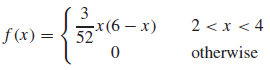×
Get Full Access to Statistics For Engineers And Scientists - 4 Edition - Chapter 2.4 - Problem 23e
Get Full Access to Statistics For Engineers And Scientists - 4 Edition - Chapter 2.4 - Problem 23e

×

# The thickness of a washer (in mm) is a random variableISBN: 9780073401331 38

## Solution for problem 23E Chapter 2.4

Statistics for Engineers and Scientists | 4th Edition

• Textbook Solutions
• 2901 Step-by-step solutions solved by professors and subject experts
• Get 24/7 help from StudySoup virtual teaching assistantsStatistics for Engineers and Scientists | 4th Edition

4 5 1 234 Reviews
15
2
Problem 23E

The thickness of a washer (in mm) is a random variable with probability density functiona. What is the probability that the thickness is less than 2.5 m?

b. What is the probability that the thickness is between 2.5 and 3.5 m?

c. Find the mean thickness.

d. Find the standard deviation σ of the thicknesses.

e. Find the probability that the thickness is within±σ of the mean.

f. Find the cumulative distribution function of the thickness.

Step-by-Step Solution:

Solution

Step 1 of 6 :

The thickness of a washer is a random variable with the probability density function isOur goal is to find :

a). What is the probability that the thickness is less than 2.5 m.

b). What is the probability that the thickness is between 2.5 and 3.5 m.

c). Find the mean thickness.

d). Find the standard deviation of the thickness.

e). Find the probability that the thickness is withinof the mean.

f).  Find the cumulative distribution function of the thickness

a).

Now we have to find the probability that the thickness is less than 2.5 m.

The probability density function isHere we take the limits 2 to 2.5.

P(x < 2.5)Here we computing the indefinite integral.

Then,

P(x < 2.5)We calculated the equation.

Then we get

P(x < 2.5)0.781252P(x < 2.5)P(x < 2.5)Therefore the probability that the thickness is less than 2.5 m is 0.2428.

Step 2 of 6

Step 3 of 6

##### ISBN: 9780073401331

The answer to “?The thickness of a washer (in mm) is a random variable with probability density function a. What is the probability that the thickness is less than 2.5 m?b. What is the probability that the thickness is between 2.5 and 3.5 m?c. Find the mean thickness.d. Find the standard deviation ? of the thicknesses.e. Find the probability that the thickness is within±? of the mean.f. Find the cumulative distribution function of the thickness.” is broken down into a number of easy to follow steps, and 72 words. Statistics for Engineers and Scientists was written by and is associated to the ISBN: 9780073401331. This full solution covers the following key subjects: thickness, Probability, Find, function, mean. This expansive textbook survival guide covers 153 chapters, and 2440 solutions. The full step-by-step solution to problem: 23E from chapter: 2.4 was answered by , our top Statistics solution expert on 06/28/17, 11:15AM. This textbook survival guide was created for the textbook: Statistics for Engineers and Scientists , edition: 4. Since the solution to 23E from 2.4 chapter was answered, more than 1874 students have viewed the full step-by-step answer.

Unlock Textbook Solution